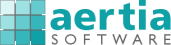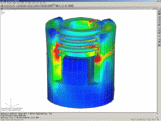http://www.aertia.com

# NEiFatigue

##### by Noran Engineering

NEiFatigue (WinLife) allows Engineers to calculate the fatigue life of components under dynamic loading. The fatigue life can be calculated by using a classical Nominal Stress method, or by using the more sophisticated Local Strain method.NEiFatigue (WinLife) allows engineers to calculate the fatigue life of components under dynamic loading. The fatigue life can be calculated by using a classical Nominal Stress method, or by using the more sophisticated Local Strain method.

Capabilities

• Fatigue Calculation without FEM
• Classical methods: nominal stress concept and local concept
• Stress S-N curves and mean stress sensitivity are required for the nominal stress concept
• Cyclic stress-strain curves, strain e-N curves and damage parameter curves according to Smith, Watson and Tooper are required for the local concept
• Loading history (stress, moment, force) obtained from a measurement can also be used
• Rainflow method used for counting of stress histories
• Easy to use Load Spectrum generator
• S-N curve generator for steel, aluminum alloys and cast iron
• Fatigue life database calculated (can be extended by the user); contains S-N curves and data describing the damage for materials commonly used
• Rainflow matrix plots
• Damage XY and Contour plots

• Fatigue Calculation Combined with FEM
• Static FE calculation is used to ascertain the stress within the component
• The direction of the standard load must correspond to the actual force
• Critical nodes can be selected for the fatigue life calculation thus reducing the calculation time
• S-N curves for failure probabilities between 0 and 100% can be created

• Multiaxial Calculation
• Method used when several loadings have an effect on a structure and are not in sync, and not proportional
• Up to 100 loadings working independently can be taken into account
• Should be used if the principal stress directions vary considerably in the component as the calculation is very time consuming
• Applications: bodywork structures, axle components, crankshafts, rotary blades for wind power stations
• Strain measurement data can be used